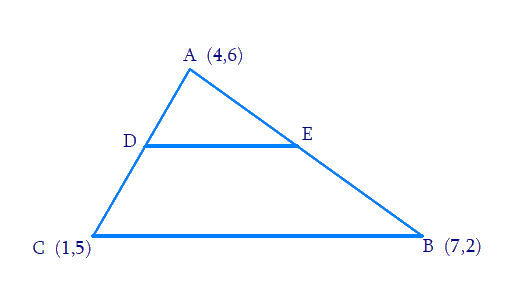# Ex.7.4 Q6 Coordinate Geometry Solution - NCERT Maths Class 10

## Question

The vertices of a $$\Delta ABC$$ are $$A \;(4, 6)$$, $$B \;(1, 5)$$ and $$C\; (7, 2)$$. $$A$$ line is drawn to intersect sides $$AB$$ and $$AC$$ at $$D$$ and $$E$$ respectively, such that \begin{align}\frac{AD}{AB} = \frac{AE}{AC} = \frac{1}{4}\,\,.\end{align}

Calculate the area of the $$\Delta ADE$$ and compare it with the area of $$\Delta ABC$$.

(Recall Converse of basic proportionality theorem and Theorem 6.6 related to Ratio of areas of two similar triangles)

Video Solution
Coordinate Geometry
Ex 7.4 | Question 6

## Text Solution

Reasoning:

Converse of Basic Proportionality Theorem: If a line divides any two sides of a triangle in the same ratio, then the line must be parallel to the third side.

Theorem $$6.6$$ : The ratio of the areas of two similar triangles is equal to the square of the ratio of their corresponding sides.

What is known?

The $$x$$ and $$y$$ coordinates of the vertices of the triangle.

\begin{align}\frac{{AD}}{{AB}} = \frac{{AE}}{{AC}} = \frac{1}{4}\end{align}

where $$AB$$ and $$AC$$ are sides of the triangle intersected at $$D$$ and $$E$$.

What is unknown?

The area of the $$\Delta ADE$$ is to be calculated and compared with the area of $$\Delta ABC$$.Steps:

From the figure,

Given

\begin{align}\frac{{AD}}{{AB}} = \frac{{AE}}{{AC}} = \frac{1}{4}\end{align}

Therefore, $$D$$ and $$E$$ are two points on side $$AB$$ and $$AC$$ respectively such that they divide side $$AB$$ and $$AC$$ in a ratio of $$1:3$$.

By Section formula

\begin{align}{{P}}(x,y) \!\!= \!\!\left[ \frac{m x_2 \!+\! n x_1}{m \!+\! n},\frac{m y_2 \!+\! n y_1}{m \!+\! n} \right] \cdots (1) \end{align}

Coordintaes of Point D

\begin{align} &\!=\!\left[ {\frac{{1 \times 1 \!+\! 3 \times 4}}{{1 \!+\! 3}},\frac{{1 \times 5 \!+\! 3 \times 6}}{{1 \!+\! 3}}} \right] \\&\!=\! \left[ {\frac{{13}}{4},\frac{{23}}{4}} \right]{\text{ }}\\ \end{align}

Coordintaes of Point E

\begin{align} &\!=\! \left[ {\frac{{1 \times 7 \!+\! 3 \times 4}}{{1 \!+\! 3}},\frac{{1 \times 2 \!+\! 3 \times 6}}{{1 \!+\! 3}}} \right] \\ &\!=\! \left[ {\frac{{19}}{4},\frac{{20}}{4}} \right] \end{align}

Area of triangle

\begin{align}\!=\!\frac{1}{2} \begin{bmatrix} x_1 \left( y_2 \!-\! y_3 \right) + x_2 \left( y_3 \!-\! y_1 \right) +\\ x_3 \left( y_1 \!-\! y_2 \right) \end{bmatrix} \cdots (2) \end{align}

By substituting the vertices $$A$$, $$D$$, $$E$$ in $$(2)$$,

Area of $$\Delta ADE$$

\begin{align} &\!=\! \frac{1}{2} \begin{bmatrix} 4\left( {\frac{{23}}{4} \!-\! \frac{{20}}{4}} \right) \!+\! \frac{{13}}{4}\left( {\frac{{20}}{4} \!-\! 6} \right) \!+\!\\ \frac{{19}}{4}\left( {6 \!-\! \frac{{23}}{4}} \right) \end{bmatrix} \\&\!=\! \frac{1}{2}\left[ {3\! -\! \frac{{13}}{4} \!+\! \frac{{19}}{{16}}} \right] \\&\!=\! \frac{1}{2}\left[ {\frac{{48 \!-\! 52\! +\! 19}}{{16}}} \right] \\ &\!=\! \frac{{15}}{{32}}{\text{ Square units}}\end{align}

Clearly, the ratio between the areas of $$\Delta ADE$$ and $$\Delta ABC$$ is $$1:16$$.

Alternatively, we know that if a line segment in a triangle divides its two sides in the same ratio, then the line segment is parallel to the third side of the triangle[Converse of Basic Proportionality Theorem]. These two triangles so formed (here $$\Delta ADE$$ and $$\Delta ABC$$) will be similar to each other.

Hence, the ratio between the areas of these two triangles will be the square of the ratio between the sides of these two triangles.[Theorem 6.6]

Therefore, ratio between the areas of $$\Delta ADE$$ and

\begin{align}\Delta {\text{ABC}}\,{\text{ = }}\,{\left( {\frac{1}{2}} \right)^2} = \frac{1}{{16}}\end{align}

Learn from the best math teachers and top your exams

• Live one on one classroom and doubt clearing
• Practice worksheets in and after class for conceptual clarity
• Personalized curriculum to keep up with school